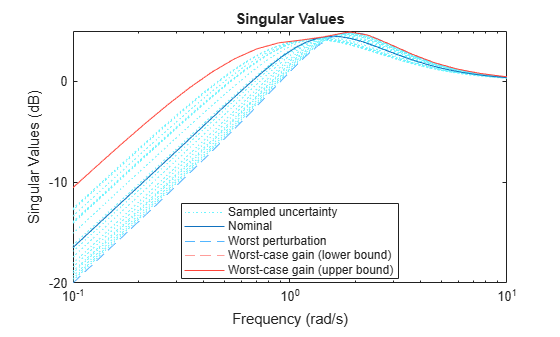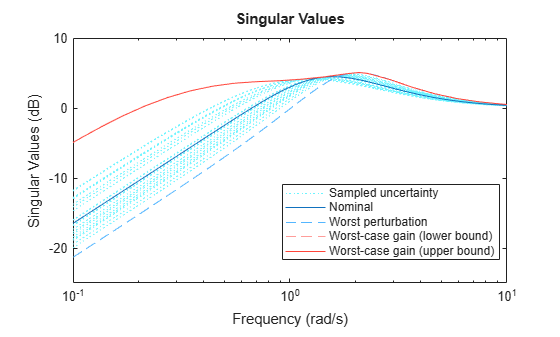# wcsigmaplot

Plot worst-case gain of uncertain system

## Syntax

``wcsigmaplot(usys)``
``wcsigmaplot(usys,w)``
``wcsigmaplot(___,opts)``

## Description

example

````wcsigmaplot(usys)` plots the nominal and worst-case gains of the uncertain system `usys` as a function of frequency. For multi-input, multi-output (MIMO) systems, gain refers to the largest singular value of the frequency response matrix. (See `sigma` for more information about singular values.) The plot includes:Nominal — Nominal gain of `usys`.Worst perturbation — The response falling within the uncertainty of `usys` that has the highest peak gain. This curve corresponds to the `wcu` output argument of `wcgain`.Worst-case gain (lower bound) — The lowest possible worst-case gain at each frequency.Worst-case gain (upper bound) — The highest possible gain within the uncertainty at each frequency. This curve represents the envelope produced by finding the highest possible gain at each frequency.Sampled Uncertainty — Responses randomly sampled from `usys`.```

example

````wcsigmaplot(usys,w)` focuses the plot on the frequencies specified by `w`.If `w` is a cell array of the form `{wmin,wmax}`, then `wcsigmaplot` plots the worst-case gains in the range `{wmin,wmax}`.If `w` is an array of frequencies, then `wcsigmaplot` plots the worst-case gains at each frequency in the array.```

example

````wcsigmaplot(___,opts)` specifies additional options for the computation. Use `wcOptions` to create `opts`.```

## Examples

collapse all

Plot the worst-case gain of the following system:

`$sys=\frac{{s}^{2}+3s}{{s}^{2}+2s+a}.$`

The uncertain parameter a = 2 $±$ 1.

```a = ureal('a',2); usys = tf([1 3 0],[1 2 a]); wcsigmaplot(usys)```The `Worst perturbation` curve identifies the single response within the uncertainty that yields the highest gain at any frequency. This perturbation corresponds to the `wcu` output of `wcgain`.

The `Worst-case gain` curves show the lower and upper bounds on the worst-case gain at each frequency. For any perturbation within the specified uncertainty range, the principal gains (singular values) of the perturbed system lie below the `Worst-case gain (upper bound)` curve. In other words, this curve is the envelope produced by finding the highest gain within the uncertainty at each frequency. For this system, the lower and upper bounds are close enough to appear identical on the plot. (See `wcgain` for more information about these bounds.)

Focus the plot on the region between 0.1 and 10 rad/s.

```w = {0.1 10}; wcsigmaplot(usys,w)```Examine the effect on the worst-case response of increasing the uncertainty range. To do this without changing the uncertainty specified in `usys`, use the `ULevel` option of `wcOptions`. This option scales the normalized uncertainty by the factor you specify. For example, examine the worst-case response a 50% greater uncertainty range.

```opts = wcOptions('ULevel',1.5); wcsigmaplot(usys,w,opts)```The plot shows that increasing the uncertainty range substantially increases the worst-case gain at low frequencies.

## Input Arguments

collapse all

Dynamic system with uncertainty, specified as a `uss`, `ufrd`, `genss`, or `genfrd` model that contains uncertain elements.

For `genss` or `genfrd` models, `wcsigmaplot` uses the current value of any tunable blocks and folds them into the known (not uncertain) part of the model.

Frequencies at which to plot worst-case gains, specified as the cell array `{wmin,wmax}` or as a vector of frequency values.

• If `w` is a cell array of the form `{wmin,wmax}`, then the function plots the worst-case gains at frequencies ranging between `wmin` and `wmax`.

• If `w` is a vector of frequencies, then the function plots the worst-case gains at each specified frequency. For example, use `logspace` to generate a row vector with logarithmically spaced frequency values.

Specify frequencies in units of rad/`TimeUnit`, where `TimeUnit` is the `TimeUnit` property of the model.

Options for worst-case computation, specified as an object you create with `wcOptions`. Setting certain options for `mussv` can improve the results of the worst-case calculation. See `wcOptions` for more information.

Example: `wcOptions('ULevel',2,'MussvOptions','m3')`

## Algorithms

`wcsigmaplot` uses `wcgain` to compute the worst-case gains. Use the `opts` argument to set options for the `wcgain` algorithm.

`wcsigmaplot` uses `usample` to compute the `Sampled Uncertainty` curves.

## Version History

Introduced in R2016b

expand all